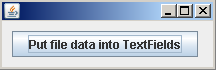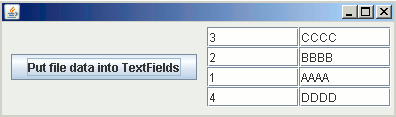# Use Map to display file data into textfields

In this section, you will learn how to store file data into HashMap and then into textfields.

In this section, you will learn how to store file data into HashMap and then into textfields.

### Use Map to display file data into textfields

Collection framework allows a programmer to store,retrieve, manipulate, and communicate aggregate data easily. You have mostly used ArrayList or Vector classes to store the file data. In this section, you will learn how to store the file data into HashMap in the form of key/value pair and then these map values have to be displayed in different textfields which are generated at run time.

In text file, we have written the following data:

 1 AAAA 2 BBBB 3 CCCC 4 DDDD

Here is the code:

```import java.io.*;
import java.util.*;
import java.awt.*;
import javax.swing.*;
import java.awt.event.*;

public class MapValuesInTextField {
public static void main(String[] args) throws Exception {
JButton b = new JButton("Put file data into TextFields");
JPanel p = new JPanel();
JPanel p1 = new JPanel();
final JPanel p2 = new JPanel(new GridLayout(4, 2));
final JFrame f = new JFrame();
f.setVisible(true);
f.pack();
public void actionPerformed(ActionEvent e) {
int i = 0;
try {
"C:/pdf.txt"));
String strLine;
String id = "", name = "";
HashMap hash = new HashMap();
while ((strLine = br.readLine()) != null) {
String[] f = strLine.split(" ");
id = f;
name = f;
hash.put(id, name);
}
Set set = hash.entrySet();
Iterator it = set.iterator();
while (it.hasNext()) {
Map.Entry me = (Map.Entry) it.next();
JTextField t1[] = new JTextField;
t1[i] = new JTextField(8);
t1[i].setText(me.getKey().toString());

JTextField t2[] = new JTextField;
t2[i] = new JTextField(8);
t2[i].setText(me.getValue().toString());
i++;
}
f.setSize(400, 120);
} catch (Exception ex) {
}
}
});
}
}
```

Output:On clicking the button, map values will get displayed in the textfields which are generated at runtime.